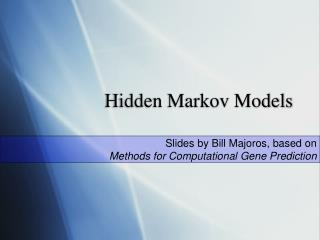DownloadDownload PresentationHidden Markov Models

# Hidden Markov Models

Télécharger la présentation## Hidden Markov Models

- - - - - - - - - - - - - - - - - - - - - - - - - - - E N D - - - - - - - - - - - - - - - - - - - - - - - - - - -
##### Presentation Transcript

1. Hidden Markov Models Slides by Bill Majoros, based on Methods for Computational Gene Prediction

2. What is an HMM? • An HMM is astochastic machineM=(Q, , Pt, Pe) consisting of the following: • a finite set of states, Q={q0, q1, ... , qm} • a finite alphabet ={s0, s1, ... , sn} • a transition distribution Pt :Q×Q a¡ i.e.,Pt (qj |qi) • an emission distribution Pe:Q×a¡ i.e.,Pe (sj|qi) An Example 5% M1=({q0,q1,q2},{Y,R},Pt,Pe) Pt={(q0,q1,1), (q1,q1,0.8), (q1,q2,0.15), (q1,q0,0.05), (q2,q2,0.7), (q2,q1,0.3)} Pe={(q1,Y,1), (q1,R,0), (q2,Y,0), (q2,R,1)} 15% Y=0% R= 100% q2 R=0% Y= 100% q1 q0 80% 30% 70% 100%

3. 5% 15% Y=0% R= 100% q2 R=0% Y= 100% q1 q0 80% 30% 70% 100% Probability of a Sequence P(YRYRY|M1) = a0→1b1,Ya1→2b2,Ra2→1b1,Ya1→2b2,Ra2→1b1,Ya1→0 =1  1  0.15  1  0.3  1  0.15  1  0.3  1  0.05 =0.00010125

4. Another Example M2= (Q, , Pt, Pe) Q = {q0, q1, q2, q3, q4}  ={A, C, G, T} q2 65% A=35% T=25% C=15% G=25% q4 q1 35% 50% A=27% T=14% C=22% G=37% A=10% T=30% C=40% G=20% 100% q0 A=11% T=17% C=43% G=29% 50% 20% 100% 80% q3

5. Finding the Most Probable Path Finding the Most Probable Path q2 65% Example: CATTAATAG A=35% T=25% C=15% G=25% q4 q1 50% 35% A=27% T=14% C=22% G=37% A=10% T=30% C=40% G=20% top: 7.0×10-7 bottom: 2.8×10-9 100% q0 A=11% T=17% C=43% G=29% 20% 50% 100% 80% q3 The most probable path is: States:122222224 Sequence: CATTAATAG resulting in this parse: States: 122222224 Sequence:CATTAATAG feature 1: C feature 2: ATTAATA feature 3: G

6. The Viterbi Algorithm . . . . . . sequence k k+1 k-2 k-1 states (i,k) . . .

7. Viterbi: Traceback T( T( T( ... T( T(i, L-1), L-2) ..., 2), 1), 0) = 0

8. The Forward Algorithm : Probability of a Sequence the single most probable path Viterbi: sum over all paths Forward: i.e., . . . . . . sequence k k+1 k-2 k-1 states (i,k) . . .

9. Training an HMM from Labeled Sequences transitions emissions From the textbook, Ch. 6.3

10. Recall: Eukaryotic Gene Structure complete mRNA coding segment ATG TGA exon intron exon intron exon . . . . . . . . . AG GT AG GT TGA ATG start codon donor site acceptor site donor site acceptor site stop codon

11. Using an HMM for Gene Prediction Intron Donor Acceptor Exon the Markov model: Start codon Stop codon Intergenic q0 the input sequence: AGCTAGCAGTATGTCATGGCATGTTCGGAGGTAGTACGTAGAGGTAGCTAGTATAGGTCGATAGTACGCGA the most probable path: the gene prediction: exon 1 exon 2 exon 3

12. Higher Order Markovian Eukaryotic Recognizer(HOMER) H17 H5 H3 H95 H27 H77

13. HOMER, version H3 Intron I=intron state E=exon state N=intergenic state Donor Acceptor  Exon Start codon Stop codon Intergenic tested on 500 Arabidopsis genes: q0

14. Recall: Sensitivity and Precision NOTE: “specificity” as defined here and throughout these slides (and the text) is really precision

15. HOMER, version H5 three exon states, for the three codon positions

16. HOMER version H17 Intron Donor Acceptor Exon Start codon Stop codon Intergenic q0 donor site acceptor site stop codon start codon

17. Maintaining Phase Across an Intron 01201201 2012012012 phase: + GTATGCGATAGTCAAGAGTGATCGCTAGACC sequence: | | | | | | | coordinates: 0 5 10 15 20 25 30

18. HOMER version H27 three separate intron models

19. Recall: Weight Matrices (stop codons) T G A (start codons) T A A A T G T A G (acceptor splice sites) (donor splice sites) A G G T

20. HOMER version H77 positional biases near splice sites

21. HOMER version H95

22. Summary of HOMER Results

23. Higher-order Markov Models P(G) A C G C T A 0th order: P(G|C) A C G C T A 1st order: P(G|AC) A C G C T A 2nd order:

24. Higher-order Markov Models 0 1 2 3 4 5

25. Summary • An HMM is a stochastic generative model which emits sequences • Parsing with an HMM can be accomplished using a decoding algorithm (such as Viterbi) to find the most probable (MAP) path generating the input sequence • Training of unambiguous HMM’s can be accomplished using labeled sequence training • Training of ambiguous HMM’s can be accomplished using Viterbi training or the Baum-Welch algorithm • Posterior decoding can be used to estimate the probability that a given symbol or substring was generate by a particular state (next lesson...)